Next: HR Threshold Up: The Late SWTCs Previous: The Late SWTCs   Contents

## The Trigger Conditions

In this section, the description of the late SWTCs is given. Then, the two cases of their application are presented separately: the on- and off-line quests.

The late SWTCs have been conceived in order to improve the background estimate and the selection criteria themselves, so that they could be applied to an on-line quest, without producing an excess of wrong detections, or distributing strongly biased peak flux or fluence estimates of true GRBs.

The most relevant improvement introduced in the background estimate of the ratemeter counts has been achieved by adopting two fitting intervals around the bin to scan, before and after it, and by performing a parabolic fit, instead of the naive average described in eq.. Let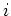th be the scanned bin and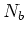and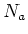the numbers of bins taken before and after theth bin, respectively (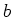=before,=after);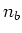andare defined so that the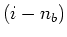th bin is the first bin of the-bin background fitting interval before the scanned bin, while the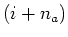th bin is the first bin of the-bin background fitting interval after the scanned bin. Let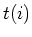and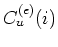be theth time bin and the counts for theth bin, for the energy band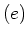and for the detector unit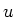; let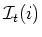be the entire time interval used for the fit and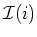its corresponding index interval. The background counts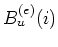are given by the 2nd order polynomial, corresponding to the least square parabolic fit of the two above intervals; to simplify the formulas in the expressions, required for calculating the 2nd order polynomial coefficients, we define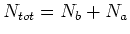and we use the notation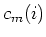, supposing thatand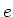are fixed. The two different sets of values used for the on- and off-line quests are reported below and will be discussed more deeply later on in this chapter. In eqq.some momenta are defined conveniently: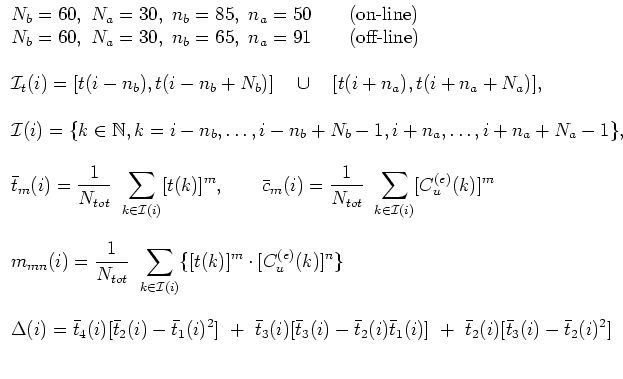(13)

According to the least square method, in order to determine the best fitting polynomial, we resolve the following set of equations: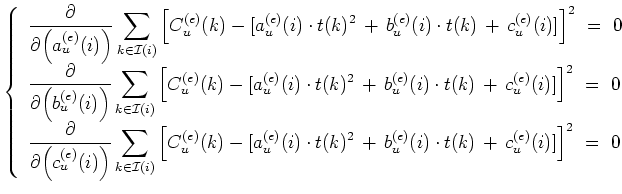(14)

The solution to the set of eqq.gives the following expressions for the coefficients of the parabolic polynomial fitting the background (eqq.)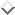: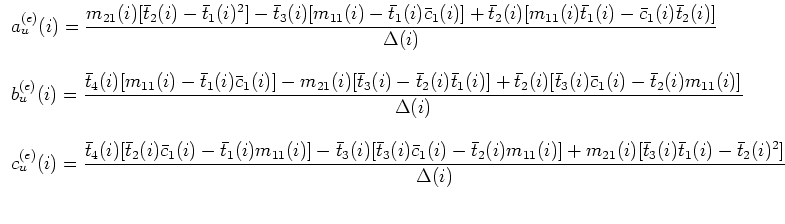(15)

Finally, the eq.gives the background counts: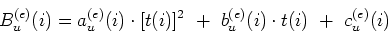(16)

In order to avoid biases of the GRB itself in the background fit, the choice of a proper value for, suitable for long duration GRBs in particular, becomes crucial. Actually, a small value forwould produce strong biases for all the bursts with time durations greater thans; on the other hand, a very big value forcould make the background fit worse, whenever the whole time interval, lasting from theth to the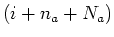th bins, would suffer from higher degree polynomial background level variations. Therefore, a proper choice, balancing the undesired effects of these two extreme possibilities, is difficult and must be adapted to the needs of the different quests.

Like in the case of the early SWTCs (see eq.), we define the net signal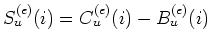and the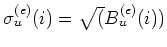. Let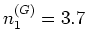,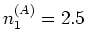,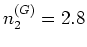,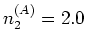,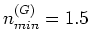,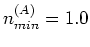,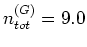,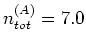, be different threshold parameters, whose meaning will be explained below, in the description of the SWTCs. At this point, all the ingredients required to express the late SWTCs are available: the SWTCs are four, in addition to a further condition on the hardness ratio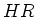defined below.

Similarly to the early SWTCs (eq.,,), the late SWTCs are expressed by the following eqq.,,,: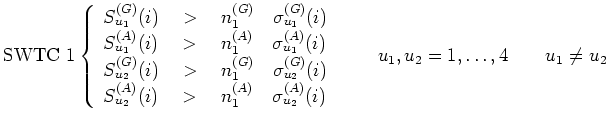(17)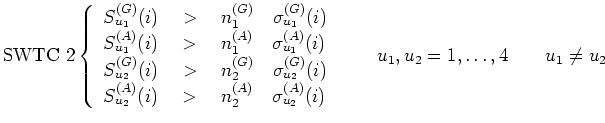(18)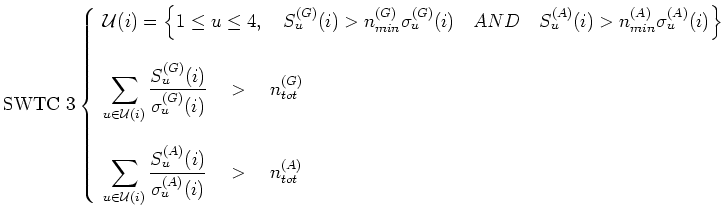(19)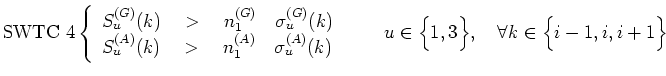(20)

The SWTC 1 (eq.) is similar to the early SWTC 1 (eq.), apart from the different values of the threshold parameters.

The SWTC 2 (eq.) is similar to the SWTC 1, with the only difference that the thresholds are no more the same for the two brightest units, but it lowers those for the second brightest one:in place ofandin place of.

The SWTC 3 (eq.) lowers the single unit thresholds and, at the same time, requires that the total sum of the net signals, expressed in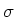, over the set of units matching the lower single unit thresholds, must be greater than a proper threshold, one for each energy band: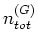and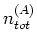.

Finally, the SWTC 4 (eq.) requires that in at least one of the GRBM units 1 and 3, the ones co-aligned with the WFCs (see fig.), the counts exceed the thresholds in both energy ranges in at least three contiguous bins.

SubsectionsNext: HR Threshold Up: The Late SWTCs Previous: The Late SWTCs   Contents
Cristiano Guidorzi 2003-07-31